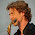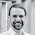+ -

## Friday, 18 March 2016

### Variography with gstat and ggplot2 18.3.16

Last year I wrote a short demo on variography with gstat and ggplot2 for a colleague who was planning to migrate to R. Just thought I’d share this here (with some additional stuff) as it might be useful for other people as well.
First, make sure you have the necessary packages installed and loaded:
``require('gstat')``
``## Loading required package: gstat``
``require('sp')``
``## Loading required package: sp``
``require('ggplot2')``
``## Loading required package: ggplot2``
The sp package is not really required, but it makes working with spatial data a little bit easier.
I like to work with the black & white ggplot2 theme, and legends positioned above the graphs, so I always adjust the basic settings the following way:
```
theme_set(theme_bw())
`theme_update(legend.position='top')````
Let’s create some synthetic data to work with. I’m using gstat here to simulate a random field on a 100x100 regular grid:
``````x <- 1:100 # x coordinates
y <- 1:100 # y coordinates
dat <- expand.grid(x=x,y=y) # create data frame with all combinations
dat\$z <- 1 # initialize z variable
coordinates(dat) <- ~x+y # set coordinates
gridded(dat) <- TRUE # specify data is gridded
g <- gstat(id='z',formula=z~1,model=vgm(0.9,'Sph',60,0.1,anis=c(45,0.1)),data=dat,dummy=TRUE,beta=0,maxdist=20,nmax=10) # create gstat object
dat <- data.frame(predict(g,newdata=dat,nsim=1)) # simulate random field data``````
``## [using unconditional Gaussian simulation]``
``````names(dat) <- 'z'
head(dat) # show first lines of the data frame``````
``````##   x y           z
## 1 1 1  1.36694227
## 2 2 1  0.58134568
## 3 3 1  0.04314338
## 4 4 1  0.24155909
## 5 5 1 -0.23566363
## 6 6 1 -0.58667780``````
Plotting data on a regular grid is easily achieved with geom_raster():
``ggplot(dat,aes(x=x,y=y,fill=z)) + geom_raster() + scale_fill_gradientn(colours=rainbow(7))``This is the data we will perform some variography on. Alternatively, have a look at this file to load your own data. We’ll transform our data frame into a spatial points data frame, and create a gstat object:
``````coordinates(dat) <- ~x+y # set coordinate variables
# gridded(dat) <- TRUE # specify that your data is gridded; this speeds up things considerably!``````
I commented the above line, as there is an issue with gstat 1.1-0, which basically mirrors gridded data horizontally before deriving the experimental variogram. Edzer Pebesma, the author of gstat, already solved the issue, so in the latest gstat releases this should work properly with gridded data as well.
``g <- gstat(id='z',formula=z~1,data=dat)``
Calculating an experimental isotropic variogram can then simply be done by:
``````expvar <- variogram(g)
head(expvar) # show first lines of the gstatVariogram data frame``````
``````##        np      dist     gamma dir.hor dir.ver id
## 1  136418  2.096697 0.3739120       0       0  z
## 2  432526  4.791096 0.6396000       0       0  z
## 3  703154  7.895251 0.8142622       0       0  z
## 4  915858 10.992501 0.8758301       0       0  z
## 5 1109122 14.046645 0.8923462       0       0  z
## 6 1327916 17.122199 0.8846601       0       0  z``````
``plot(expvar) # you can plot gstatVariogram objects like this (gstat function)``I prefer ggplot2 for plotting, and it turns out that the gstat objects are very much suited for use with ggplot2:
``ggplot(expvar,aes(x=dist,y=gamma,size=np)) + geom_point()``For a single direction at a time, you can just specify the angle alpha and the tolerance on the angle:
``````expvar <- variogram(g,alpha=0,tol.hor=5)
ggplot(expvar,aes(x=dist,y=gamma,size=np)) + geom_point()``````Looking at multiple directions is done by providing a vector instead of a single value:
``````expvar <- variogram(g,alpha=c(0,45,90,135),tol.hor=5)
ggplot(expvar,aes(x=dist,y=gamma,size=np,col=factor(dir.hor))) + geom_point()``````And cutoff values can be specified as well if the default value does not suffice:
``````expvar <- variogram(g,alpha=c(0,45,90,135),tol.hor=5,cutoff=30)
ggplot(expvar,aes(x=dist,y=gamma,size=np,col=factor(dir.hor))) + geom_point()``````Another option is to create a variogram map:
``expvar <- variogram(g,width=3,cutoff=30,map=TRUE)``
which can be plotted again by a gstat function:
``plot(expvar)``The variogram map can again easily be plotted with ggplot2 and geom_raster():
``ggplot(data.frame(expvar),aes(x=map.dx,y=map.dy,fill=map.z))+geom_raster() + scale_fill_gradientn(colours=rainbow(7))``Finally, my colleague was interested in the performance of the algorithm. So here are some execution times for my personal laptop, running Linux Lite 2.6, R 3.2.3, and having an Intel(R) Core(TM)2 Duo CPU T5800 @ 2.00GHz processor and 2969MB memory:
``system.time(variogram(g))``
``````##    user  system elapsed
##   5.192   0.072   7.698``````
``system.time(variogram(g,alpha=0,tol.hor=5))``
``````##    user  system elapsed
##   4.828   0.104   6.553``````
``system.time(variogram(g,alpha=c(0,45,90,135),tol.hor=5))``
``````##    user  system elapsed
##  19.240   0.440  26.115``````
``system.time(variogram(g,alpha=c(0,45,90,135),tol.hor=5,cutoff=100))``
``````##    user  system elapsed
##  29.040   0.680  40.121``````
That’s it! If you want to know more, have a look at
``?variogram``
for more options, and if you want to try this yourself, check out the source R script!

Update 11.4.16
Here are some more examples to provide an answer to Edzer’s two very relevant comments. Including the origin is straightforward with expand_limits():
``````load('expvar.rda')
ggplot(expvar,aes(x=dist,y=gamma,size=np,col=factor(dir.hor))) + geom_point() + expand_limits(x = 0, y = 0)``````For drawing the attention to the behaviour near the origin, I see several possibilities. One is to use the size aesthetic for the weights you would use for variogram fitting. Here’s what happens with the weights of the default fit method of fit.variogram:
``ggplot(expvar,aes(x=dist,y=gamma,size=np/dist^2,col=factor(dir.hor))) + geom_point() + expand_limits(x = 0, y = 0)``Luckily, other aesthetics are also available for geom_point(). I find it quite intuitive to use the size for the number of points, so using transparency to illustrate the weight for the different points results in:
``ggplot(expvar,aes(x=dist,y=gamma,size=np,alpha=log10(np/dist^2),col=factor(dir.hor))) + geom_point() + expand_limits(x = 0, y = 0)``This seems to be my favorite solution, but with the additional shape, fill and stroke aesthetics, many different possibilities are of course available. Here’s another interesting one (although stroke legends seem to be problematic):
``ggplot(expvar,aes(x=dist,y=gamma,size=np/dist^2,fill=factor(dir.hor),stroke=10*np/max(np))) + geom_point(shape=21,colour='lightgray') + expand_limits(x = 0, y = 0)``5 Bart Rogiers: Variography with gstat and ggplot2 Last year I wrote a short demo on  variography  with  gstat  and  ggplot2  for a colleague who was planning to migrate to  R . Just though...

1.Nice blog. Two comments: (i) variogram plot y axes always start at 0, for the same reason that autocorrelation diagrams will start at 1; (ii) showing symbol size proportional to Nh draws the attention away from the essential information: behaviour near the origin. Can you given an example how to resolve (i)?

1.Thanks for the useful comments Edzer! I just included a small update. Hope you like it!

2.Hi,
Nice post. I came across here while trying to ggplot a fit.variogram object. gstat variogram object goes nicely with ggplot but I can't really say the same thing for fit.variogram object

1.Thanks! You should check out ?variogramLine for that!